# 9.6: Solve Equations with Square Roots

•• OpenStax
• OpenStax
$$\newcommand{\vecs}{\overset { \rightharpoonup} {\mathbf{#1}} }$$ $$\newcommand{\vecd}{\overset{-\!-\!\rightharpoonup}{\vphantom{a}\smash {#1}}}$$$$\newcommand{\id}{\mathrm{id}}$$ $$\newcommand{\Span}{\mathrm{span}}$$ $$\newcommand{\kernel}{\mathrm{null}\,}$$ $$\newcommand{\range}{\mathrm{range}\,}$$ $$\newcommand{\RealPart}{\mathrm{Re}}$$ $$\newcommand{\ImaginaryPart}{\mathrm{Im}}$$ $$\newcommand{\Argument}{\mathrm{Arg}}$$ $$\newcommand{\norm}{\| #1 \|}$$ $$\newcommand{\inner}{\langle #1, #2 \rangle}$$ $$\newcommand{\Span}{\mathrm{span}}$$ $$\newcommand{\id}{\mathrm{id}}$$ $$\newcommand{\Span}{\mathrm{span}}$$ $$\newcommand{\kernel}{\mathrm{null}\,}$$ $$\newcommand{\range}{\mathrm{range}\,}$$ $$\newcommand{\RealPart}{\mathrm{Re}}$$ $$\newcommand{\ImaginaryPart}{\mathrm{Im}}$$ $$\newcommand{\Argument}{\mathrm{Arg}}$$ $$\newcommand{\norm}{\| #1 \|}$$ $$\newcommand{\inner}{\langle #1, #2 \rangle}$$ $$\newcommand{\Span}{\mathrm{span}}$$$$\newcommand{\AA}{\unicode[.8,0]{x212B}}$$

##### Learning Objectives

By the end of this section, you will be able to:

• Use square roots in applications
##### Note

Before you get started, take this readiness quiz.

1. Simplify: ⓐ $$\sqrt{9}$$ ⓑ $$9^2$$.
If you missed this problem, review Example 9.1.1 and Exercise 1.3.22.
2. Solve: 5(x+1)−4=3(2x−7).
If you missed this problem, review Exercise 2.4.16.
3. Solve: $$n^2−6n+8=0$$.
If you missed this problem, review Exercise 7.6.13.

In this section we will solve equations that have the variable in the radicand of a square root. Equations of this type are called radical equations.

An equation in which the variable is in the radicand of a square root is called a radical equation.

As usual, in solving these equations, what we do to one side of an equation we must do to the other side as well. Since squaring a quantity and taking a square root are ‘opposite’ operations, we will square both sides in order to remove the radical sign and solve for the variable inside.

But remember that when we write $$\sqrt{a}$$ we mean the principal square root. So $$\sqrt{a} \ge 0$$ always. When we solve radical equations by squaring both sides we may get an algebraic solution that would make $$\sqrt{a}$$ negative. This algebraic solution would not be a solution to the original radical equation; it is an extraneous solution. We saw extraneous solutions when we solved rational equations, too.

##### Example $$\PageIndex{1}$$

For the equation $$\sqrt{x+2}=x$$:

1. Is x=2 a solution?
2. Is x=−1 a solution?

1. Is x=2 a solution?Let x = 2.Simplify.2 is a solution.

2. Is x=−1 a solution?Let x = −1.Simplify.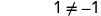−1 is not a solution. −1 is an extraneous solution to the equation.
##### Example $$\PageIndex{2}$$

For the equation $$\sqrt{x+6}=x$$:

1. Is x=−2 a solution?
2. Is x=3 a solution?
1. no
2. yes
##### Example $$\PageIndex{3}$$

For the equation $$\sqrt{−x+2}=x$$:

1. Is x=−2 a solution?
2. Is x=1 a solution?
1. no
2. yes
Now we will see how to solve a radical equation. Our strategy is based on the relation between taking a square root and squaring.

For$$a \ge 0$$, $$(\sqrt{a})^2=a$$

##### Example $$\PageIndex{4}$$

Solve: $$\sqrt{2x−1}=7$$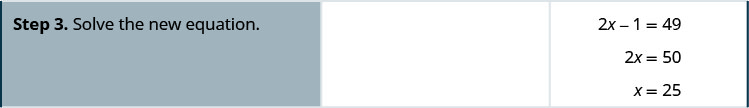##### Example $$\PageIndex{5}$$

Solve: $$\sqrt{3x−5}=5$$.

10

##### Example $$\PageIndex{6}$$

Solve: $$\sqrt{4x+8}=6$$.

7

##### Definition: SOLVE A RADICAL EQUATION.
1. Isolate the radical on one side of the equation.
2. Square both sides of the equation.
3. Solve the new equation.
##### Example $$\PageIndex{7}$$

Solve: $$\sqrt{5n−4}−9=0$$.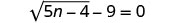To isolate the radical, add 9 to both sides.Simplify.Square both sides of the equation.Solve the new equation.Check the answer.The solution is n = 17.
##### Example $$\PageIndex{8}$$

Solve: $$\sqrt{3m+2}−5=0$$.

$$\frac{23}{3}$$

##### Example $$\PageIndex{9}$$

Solve: $$\sqrt{10z+1}−2=0$$.

$$\frac{3}{10}$$

##### Example $$\PageIndex{10}$$

Solve: $$\sqrt{3y+5}+2=5$$.To isolate the radical, subtract 2 from both sides.Simplify.Square both sides of the equation.Solve the new equation.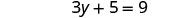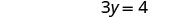Check the answer.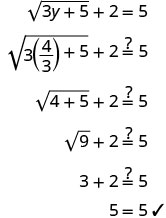The solution is $$y=\frac{4}{3}$$
##### Example $$\PageIndex{11}$$

Solve: $$\sqrt{3p+3}+3=5$$.

$$\frac{1}{3}$$

##### Example $$\PageIndex{12}$$

Solve: $$\sqrt{5q+1}+4=6$$.

$$\frac{3}{5}$$

When we use a radical sign, we mean the principal or positive root. If an equation has a square root equal to a negative number, that equation will have no solution.

##### Example $$\PageIndex{13}$$

Solve: $$\sqrt{9k−2}+1=0$$.To isolate the radical, subtract 1 from both sides.Simplify.Since the square root is equal to a negative number, the equation has no solution.
##### Example $$\PageIndex{14}$$

Solve: $$\sqrt{2r−3}+5=0$$

no solution

##### Example $$\PageIndex{15}$$

Solve: $$\sqrt{7s−3}+2=0$$.

no solution

If one side of the equation is a binomial, we use the binomial squares formula when we square it.
##### Definition: BINOMIAL SQUARES

$\begin{array}{cc} {(a+b)^2=a^2+2ab+b^2}&{(a−b)^2=a^2−2ab+b^2}\\ \nonumber \end{array}$

Don’t forget the middle term!

##### Example $$\PageIndex{16}$$

Solve: $$\sqrt{p−1}+1=p$$.To isolate the radical, subtract 1 from both sides.Simplify.Square both sides of the equation.Simplify, then solve the new equation.It is a quadratic equation, so get zero on one side.Factor the right side.Use the zero product property.Solve each equation.Check the answers.The solutions are p = 1, p = 2.
##### Example $$\PageIndex{17}$$

Solve: $$\sqrt{x−2}+2=x$$.

2, 3

##### Example $$\PageIndex{18}$$

Solve: $$\sqrt{y−5}+5=y$$.

5, 6

##### Example $$\PageIndex{19}$$

Solve: $$\sqrt{r+4}−r+2=0$$.

 $$\sqrt{r+4}−r+2=0$$ Isolate the radical. $$\sqrt{r+4}=r−2$$ Square both sides of the equation. $$(\sqrt{r+4})^2=(r−2)^2$$ Solve the new equation. $$r+4=r^2−4r+4$$ It is a quadratic equation, so get zero on one side. $$0=r^2−5r$$ Factor the right side. $$0=r(r−5)$$ Use the zero product property. 0=r 0=r−5 Solve the equation. r=0 r=5 Check the answer.r=5 r=0 is an extraneous solution.
##### Example $$\PageIndex{20}$$

Solve: $$\sqrt{m+9}−m+3=0$$.

7

##### Example $$\PageIndex{21}$$

Solve: $$\sqrt{n+1}−n+1=0$$

3

When there is a coefficient in front of the radical, we must square it, too.

##### Example $$\PageIndex{22}$$

Solve: $$3\sqrt{3x−5}−8=4$$.

 $$3\sqrt{3x−5}−8=4$$ Isolate the radical. $$3\sqrt{3x−5}=12$$ Square both sides of the equation. $$(3\sqrt{3x−5})^2=(12)^2$$ Simplify, then solve the new equation. 9(3x−5)=144 Distribute. 27x−45=144 Solve the equation. 27x=189 x=7 Check the answer.The solution is x=7.
##### Example $$\PageIndex{23}$$

Solve: $$\sqrt{24a+2}−16=16$$.

$$\frac{127}{2}$$

##### Example $$\PageIndex{24}$$

Solve: $$\sqrt{36b+3}−25=50$$.

$$\frac{311}{3}$$

##### Example $$\PageIndex{25}$$

Solve: $$\sqrt{4z−3}=\sqrt{3z+2}$$.

 $$\sqrt{4z−3}=\sqrt{3z+2}$$ The radical terms are isolated $$\sqrt{4z−3}=\sqrt{3z+2}$$ Square both sides of the equation. $$(\sqrt{4z−3})^2=(\sqrt{3z+2})^2$$ Simplify, then solve the new equation 4z−3=3z+2 z−3=2 z=5 x=7 Check the answer. We leave it to you to show that 5 checks! The solution is z=5.
##### Example $$\PageIndex{26}$$

Solve: $$\sqrt{2x−5}=\sqrt{5x+3}$$.

no solution

##### Example $$\PageIndex{27}$$

Solve: $$\sqrt{7y+1}=\sqrt{2y−5}$$.

no solution

Sometimes after squaring both sides of an equation, we still have a variable inside a radical. When that happens, we repeat Step 1 and Step 2 of our procedure. We isolate the radical and square both sides of the equation again.

##### Example $$\PageIndex{28}$$

Solve: $$\sqrt{m}+1=\sqrt{m+9}$$.

 $$\sqrt{m}+1=\sqrt{m+9}$$ The radical on the right side is isolated. Square both sides $$(\sqrt{m}+1)^2=(\sqrt{m+9})^2$$ Simplify—be very careful as you multiply! $$m+2\sqrt{m}+1=m+9$$ There is still a radical in the equation. So we must repeat the previous steps. Isolate the radical. $$2\sqrt{m}=8$$ Square both sides. $$(2\sqrt{m})^2=(8)^2$$ Simplify, then solve the new equation. 4m=64 m=16 Check the answer. We leave it to you to show that m=16 checks! The solution is m=16.
##### Example $$\PageIndex{29}$$

Solve: $$\sqrt{x}+3=\sqrt{x+5}$$.

no solution

##### Example $$\PageIndex{30}$$

Solve: $$\sqrt{m}+5=\sqrt{m+16}$$.

no solution

##### Example $$\PageIndex{31}$$

Solve: $$\sqrt{q−2}+3=\sqrt{4q+1}$$.

 $$\sqrt{q−2}+3=\sqrt{4q+1}$$ The radical on the right side is isolated. Square both sides $$(\sqrt{q−2}+3)^2=(\sqrt{4q+1})^2$$ Simplify. $$q−2+6\sqrt{q−2}+9=4q+1$$ There is still a radical in the equation. So we must repeat the previous steps. Isolate the radical. $$6\sqrt{q−2}=3q−6$$ Square both sides. $$(6\sqrt{q−2})^2=(3q−6)^2$$ Simplify, then solve the new equation. $$36(q−2)=9q^2−36q+36$$ Distribute. $$36q−72=9q^2−36q+36$$ It is a quadratic equation, so get zero on one side. $$0=9q^2−72q+108$$ Factor the right side. $$0=9(q^2−8q+12)$$ $$0=9(q−6)(q−2)$$ Use the zero product property $\begin{array}{ll} {q−6=0}&{q−2=0}\\ {q=6}&{q=2}\\ \nonumber \end{array}$ The checks are left to you. (Both solutionsshould work.) The solutions are q=6 and q=2.
##### Example $$\PageIndex{32}$$

Solve: $$\sqrt{y−3}+2=\sqrt{4y+2}$$.

no solution​​​​​​​

##### Example $$\PageIndex{33}$$

Solve: $$\sqrt{n−4}+5=\sqrt{3n+3}$$.

no solution

## Use Square Roots in Applications

As you progress through your college courses, you’ll encounter formulas that include square roots in many disciplines. We have already used formulas to solve geometry applications.

We will use our Problem Solving Strategy for Geometry Applications, with slight modifications, to give us a plan for solving applications with formulas from any discipline.

##### Definition: SOLVE APPLICATIONS WITH FORMULAS.
1. Read the problem and make sure all the words and ideas are understood. When appropriate, draw a figure and label it with the given information.
2. Identify what we are looking for.
3. Name what we are looking for by choosing a variable to represent it.
4. Translate into an equation by writing the appropriate formula or model for the situation. Substitute in the given information.
5. Solve the equation using good algebra techniques.
6. Check the answer in the problem and make sure it makes sense.
7. Answer the question with a complete sentence.

​​​​​​​We used the formula A=L·W to find the area of a rectangle with length L and width W. A square is a rectangle in which the length and width are equal. If we let s be the length of a side of a square, the area of the square is $$s^2$$.The formula $$A=s^2$$ gives us the area of a square if we know the length of a side. What if we want to find the length of a side for a given area? Then we need to solve the equation for s.

$\begin{array}{ll} {}&{A=s^2}\\ {\text{Take the square root of both sides.}}&{\sqrt{A}=\sqrt{s^2}}\\ {\text{Simplify.}}&{s=\sqrt{A}}\\ \nonumber \end{array}$

We can use the formula $$s=\sqrt{A}$$ to find the length of a side of a square for a given area.

##### Definition: AREA OF A SQUARE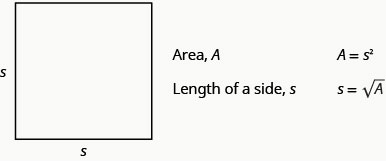​​​​​​​We will show an example of this in the next example.

##### Example $$\PageIndex{34}$$

Mike and Lychelle want to make a square patio. They have enough concrete to pave an area of 200 square feet. Use the formula $$s=\sqrt{A}$$ to find the length of each side of the patio. Round your answer to the nearest tenth of a foot.

 Step 1. Read the problem. Draw a figure and label it with the given information.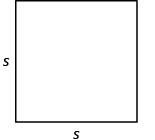A = 200 square feet Step 2. Identify what you are looking for. The length of a side of the square patio. Step 3. Name what you are looking for by choosing a variable to represent it. Let s = the length of a side. Step 4. Translate into an equation by writing the appropriate formula or model for the situation. Substitute the given information.Step 5. Solve the equation using good algebra techniques. Round to one decimal place.Step 6. Check the answer in the problem and make sure it makes sense.This is close enough because we rounded the square root. Is a patio with side 14.1 feet reasonable? Yes. Step 7. Answer the question with a complete sentence. Each side of the patio should be 14.1 feet.
##### Example $$\PageIndex{35}$$

Katie wants to plant a square lawn in her front yard. She has enough sod to cover an area of 370 square feet. Use the formula $$s=\sqrt{A}$$ to find the length of each side of her lawn. Round your answer to the nearest tenth of a foot.

19.2 feet

##### Example $$\PageIndex{36}$$

Sergio wants to make a square mosaic as an inlay for a table he is building. He has enough tile to cover an area of 2704 square centimeters. Use the formula $$s=\sqrt{A}$$ to find the length of each side of his mosaic. Round your answer to the nearest tenth of a foot.

52.0 cm

​​​​​​​Another application of square roots has to do with gravity.

##### Definition: FALLING OBJECTS

On Earth, if an object is dropped from a height of hh feet, the time in seconds it will take to reach the ground is found by using the formula,​​​​​​​

$$t=\frac{\sqrt{h}}{4}$$

​​​​​​​For example, if an object is dropped from a height of 64 feet, we can find the time it takes to reach the ground by substituting h=64 into the formula.Take the square root of 64.Simplify the fraction.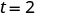It would take 2 seconds for an object dropped from a height of 64 feet to reach the ground.

##### Example $$\PageIndex{37}$$

Christy dropped her sunglasses from a bridge 400 feet above a river. Use the formula $$t=\frac{\sqrt{h}}{4}$$ to find how many seconds it took for the sunglasses to reach the river.

 Step 1. Read the problem. Step 2. Identify what you are looking for. The time it takes for the sunglasses to reach the river. Step 3. Name what you are looking for by choosing a variable to represent it. Let t = time. Step 4. Translate into an equation by writing the appropriate formula or model for the situation. Substitute in the given information.Step 5. Solve the equation using good algebra techniques.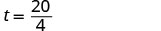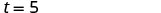Step 6. Check the answer in the problem and make sure it makes sense.5=5✓ Does 5 seconds seem reasonable? Yes. Step 7. Answer the question with a complete sentence. It will take 5 seconds for the sunglasses to hit the water.​​​​​​​
##### Exercise $$\PageIndex{38}$$

A helicopter dropped a rescue package from a height of 1,296 feet. Use the formula $$t=\frac{\sqrt{h}}{4}$$ to find how many seconds it took for the package to reach the ground.

9 seconds

##### Example $$\PageIndex{39}$$

A window washer dropped a squeegee from a platform 196 feet above the sidewalk Use the formula $$t=\frac{\sqrt{h}}{4}$$ to find how many seconds it took for the squeegee to reach the sidewalk.

3.5 seconds

Police officers investigating car accidents measure the length of the skid marks on the pavement. Then they use square roots to determine the speed, in miles per hour, a car was going before applying the brakes.

##### Definition: SKID MARKS AND SPEED OF A CAR

If the length of the skid marks is d feet, then the speed, s, of the car before the brakes were applied can be found by using the formula,

$$s=\sqrt{24d}$$​​​​​​​

##### Example $$\PageIndex{40}$$

After a car accident, the skid marks for one car measured 190 feet. Use the formula $$s=\sqrt{24d}$$ to find the speed of the car before the brakes were applied. Round your answer to the nearest tenth.

 Step 1. Read the problem. Step 2. Identify what we are looking for. The speed of a car. Step 3. Name what we are looking for. Let s = the speed. Step 4. Translate into an equation by writing the appropriate formula.Substitute the given information.Step 5. Solve the equation.Round to 1 decimal place.Step 6. Check the answer in the problem. 67.5≈?24(190)‾‾‾‾‾‾‾‾√ 67.5≈?4560‾‾‾‾‾√ 67.5≈?67.5277... Is 67.5 mph a reasonable speed? Yes. Step 7. Answer the question with a complete sentence. The speed of the car was approximately 67.5 miles per hour.
##### Example $$\PageIndex{41}$$

An accident investigator measured the skid marks of the car. The length of the skid marks was 76 feet. Use the formula $$s=\sqrt{24d}$$ to find the speed of the car before the brakes were applied. Round your answer to the nearest tenth.

42.7 feet

##### Example $$\PageIndex{42}$$

The skid marks of a vehicle involved in an accident were 122 feet long. Use the formula $$s=\sqrt{24d}$$ to find the speed of the vehicle before the brakes were applied. Round your answer to the nearest tenth.

54.1 feet

## Key Concepts

• To Solve a Radical Equation:
1. Isolate the radical on one side of the equation.
2. Square both sides of the equation.
3. Solve the new equation.
4. Check the answer. Some solutions obtained may not work in the original equation.
• Solving Applications with Formulas
1. Read the problem and make sure all the words and ideas are understood. When appropriate, draw a figure and label it with the given information.
2. Identify what we are looking for.
3. Name what we are looking for by choosing a variable to represent it.
4. Translate into an equation by writing the appropriate formula or model for the situation. Substitute in the given information.
5. Solve the equation using good algebra techniques.
6. Check the answer in the problem and make sure it makes sense.
7. Answer the question with a complete sentence.
• Area of a Square• Falling Objects
• On Earth, if an object is dropped from a height of hh feet, the time in seconds it will take to reach the ground is found by using the formula$$t=\frac{\sqrt{h}}{4}$$.
• Skid Marks and Speed of a Car
• If the length of the skid marks is d feet, then the speed, s, of the car before the brakes were applied can be found by using the formula $$s=\sqrt{24d}$$.